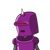# convert the following speed as a pur given: 72km/hour into m/sec the answer is 20 m/sec​

convert the following speed as a pur given: 72km/hour into m/sec the answer is 20 m/sec​

### 1 thought on “convert the following speed as a pur given: 72km/hour into m/sec the answer is 20 m/sec​”

1.20m/sec

Step-by-step explanation:

Solution

1km/hour = 1000m/60×60sec

so 72km/hour = 72×1000/60×60

=> 20m/sec

Hence , 72km/hour in m/sec is 20m/sec